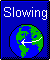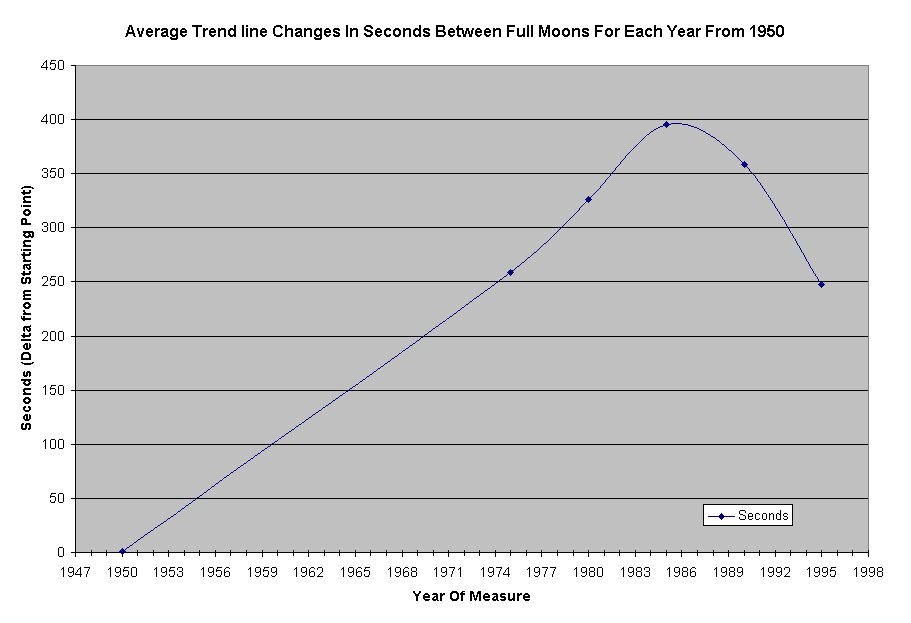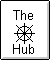#Full Moon Trend

The published date and time (nearest Minute) for Full moons from 1950 to present time (2001) is tabulated by the Navy. Because of the amount of variation in data points from year to year, a large number of points was needed to get a good average and see the current trends.

The trend for the last 52 years (1950-2001) is shown as a 10.3 sec/year slope.
The trend for the last 42 years (1960-2001) is shown as a 13.6 sec/year slope.
The trend for the last 32 years (1970-2001) is shown as a 13.8 sec/year slope.
The trend for the last 22 years (1980-2001) is shown as a -7.4 sec/year slope.
The trend for the last 12 years (1990-2001) is shown as a -22.3 sec/year slope.

If one now takes the left half of each trend line and strings them together for the appropriate number of years (that they would apply to) then one gets an overall trend chart. This curve changed to seconds (of change) from year to year then gives the chart below.We observed the moon to start to speed up after 1985 (makes for shorter moon months), what does this mean? Assume for now the reported date-time is accurate Atomic time and this is clicking at a constant rate. Assume for now no hidden leap seconds. For a full moon the moon is measured in relation to the sun and the earth's rotation around the sun. The earth and moon has to lie along the same radial line from the sun to get a full moon. The daily rotation of the earth would then be factored out of the equation. In fact full moons could occur any time of the day. So the bottom line would be either the moon is going around the earth faster or the earth is going around the sun slower. Since we found evidence in studying Equinox data that the earth's yearly rotation around the sun is slowing then this becomes the more likely logical conclusion.

Now if one assumes the atomic clocks are being adjusted slower (added undocumented leap sec) to match a slowing daily rotation of earth then what do we get? One would expect fewer turns of the planet between full moons. This would record as shorter times between full moons, and look like a speed up of the moons rotation around the earth. This is exactly what we are seeing in the data since about 1985. 10 years later in 1995 the moons orbital rotation is about 150 sec faster for every 29.5 days. This would be an equivalent of about 30 min/year. This is a significant amount of time if Atomic Time was adjusted for. I think we would have noticed this. So I don't think this is the total answer. Are there any other conditions that could explain the moon's apparent speed up? What if gravity between the sun and the earth is weaker due to the approach of the 12th. This would be a kind of conservation of gravity type effect. More objects to pull on, results in slightly less pull for any one object. If gravity were weaker then the earth would move to a slightly larger diameters orbit. The larger orbit could take longer to get fully around one turn. Thus resulting in the measured slowing effect. Now if earth and moon is further away from the sun then the moon being in close proximity can move in closer to the earth's now stronger pull. Moving in closer allows the moon to go faster around the earth. This paragraph is just potential speculation on my part. The actual truth of what we are seeing in this data is not totally known to me at this time. It could even be a combination of the above. Additional factor are even possible.

Note: Someone is going to ask why not plot past 1995 up to 2001. The answer is not enough data points to make a good trend. 12 years is about a minimum with such widely varying data. Even that is pushing it. There are naturally long time cycles in this data. The main point with all this is there is something going on. More exact data is needed and it is worth looking at now to determine exactly what is happing. This is the challenge I give to the scientific community.

Offered by Mike.